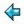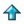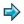### topsort.m

```topsort :: [(*,*)] -> [*]
topsort rel = tsort (carrier rel) rel

tsort c r = [], if c=[]
= error "inconsistent data for tsort",       if m=[]
= a : tsort (c--[a]) [(u,v)|(u,v)<-r; u~=a], otherwise
where
a = hd m
m = (c -- ran r)
||remarks on the above
|| - it is an invariant that c contains the carrier of relation r
|| - m is the set of elements of c with no predecessor in r

dom r = mkset [u|(u,v)<-r]  ||domain of a relation
ran r = mkset [v|(u,v)<-r]  ||range of a relation
carrier r = union (dom r) (ran r)
union x y = mkset (x++y)
```

Miranda script for topological sort

topsort takes a list of pairs representing a partial order - where the presence of (u,v) in the list means that u precedes v in the ordering - and returns a total ordering consistent with the information given - that is if (u,v) is in the input data, then u will come before v in the output list.

The error case will arise if the input data contains a cycle - i.e. if there is an element that directly or indirectly precedes itself.

A set is here represented as a list without duplicates. The standard function mkset removes duplicates from a list. The carrier of a relation is the set of all the elements related by it.